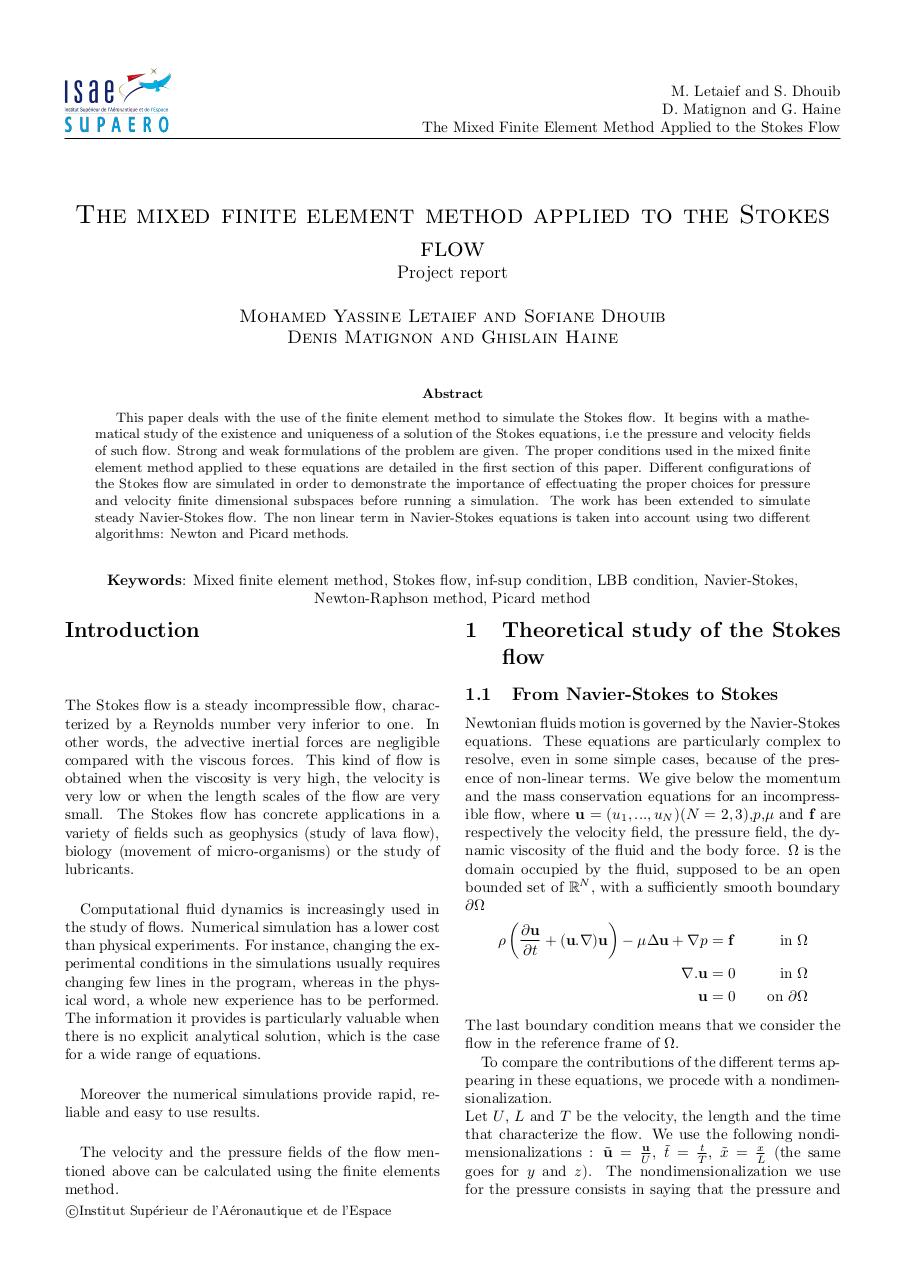# rapport (1).pdfPage 1 2 3 4 5 6 7 8

#### Text preview

M. Letaief and S. Dhouib
D. Matignon and G. Haine
The Mixed Finite Element Method Applied to the Stokes Flow

The mixed finite element method applied to the Stokes
flow
Project report

Mohamed Yassine Letaief and Sofiane Dhouib
Denis Matignon and Ghislain Haine
Abstract
This paper deals with the use of the finite element method to simulate the Stokes flow. It begins with a mathematical study of the existence and uniqueness of a solution of the Stokes equations, i.e the pressure and velocity fields
of such flow. Strong and weak formulations of the problem are given. The proper conditions used in the mixed finite
element method applied to these equations are detailed in the first section of this paper. Different configurations of
the Stokes flow are simulated in order to demonstrate the importance of effectuating the proper choices for pressure
and velocity finite dimensional subspaces before running a simulation. The work has been extended to simulate
steady Navier-Stokes flow. The non linear term in Navier-Stokes equations is taken into account using two different
algorithms: Newton and Picard methods.

Keywords: Mixed finite element method, Stokes flow, inf-sup condition, LBB condition, Navier-Stokes,
Newton-Raphson method, Picard method

Introduction

The Stokes flow is a steady incompressible flow, characterized by a Reynolds number very inferior to one. In
other words, the advective inertial forces are negligible
compared with the viscous forces. This kind of flow is
obtained when the viscosity is very high, the velocity is
very low or when the length scales of the flow are very
small. The Stokes flow has concrete applications in a
variety of fields such as geophysics (study of lava flow),
biology (movement of micro-organisms) or the study of
lubricants.
Computational fluid dynamics is increasingly used in
the study of flows. Numerical simulation has a lower cost
than physical experiments. For instance, changing the experimental conditions in the simulations usually requires
changing few lines in the program, whereas in the physical word, a whole new experience has to be performed.
The information it provides is particularly valuable when
there is no explicit analytical solution, which is the case
for a wide range of equations.
Moreover the numerical simulations provide rapid, reliable and easy to use results.
The velocity and the pressure fields of the flow mentioned above can be calculated using the finite elements
method.
c
Institut
Sup´erieur de l’A´eronautique et de l’Espace

1
1.1

Theoretical study of the Stokes
flow
From Navier-Stokes to Stokes

Newtonian fluids motion is governed by the Navier-Stokes
equations. These equations are particularly complex to
resolve, even in some simple cases, because of the presence of non-linear terms. We give below the momentum
and the mass conservation equations for an incompressible flow, where u = (u1 , ..., uN )(N = 2, 3),p,µ and f are
respectively the velocity field, the pressure field, the dynamic viscosity of the fluid and the body force. Ω is the
domain occupied by the fluid, supposed to be an open
bounded set of RN , with a sufficiently smooth boundary
∂Ω


∂u
ρ
+ (u.∇)u − µ∆u + ∇p = f
in Ω
∂t
∇.u = 0

in Ω

u=0

on ∂Ω

The last boundary condition means that we consider the
flow in the reference frame of Ω.
To compare the contributions of the different terms appearing in these equations, we procede with a nondimensionalization.
Let U , L and T be the velocity, the length and the time
that characterize the flow. We use the following nondix
˜ = Uu , t˜ = Tt , x
mensionalizations : u
˜ = L
(the same
goes for y and z). The nondimensionalization we use
for the pressure consists in saying that the pressure and